Important Questions: Factorisation

# Important Questions: Factorisation Notes | Study Mathematics (Maths) Class 8 - Class 8

## Document Description: Important Questions: Factorisation for Class 8 2022 is part of Factorisation for Mathematics (Maths) Class 8 preparation. The notes and questions for Important Questions: Factorisation have been prepared according to the Class 8 exam syllabus. Information about Important Questions: Factorisation covers topics like and Important Questions: Factorisation Example, for Class 8 2022 Exam. Find important definitions, questions, notes, meanings, examples, exercises and tests below for Important Questions: Factorisation.

Introduction of Important Questions: Factorisation in English is available as part of our Mathematics (Maths) Class 8 for Class 8 & Important Questions: Factorisation in Hindi for Mathematics (Maths) Class 8 course. Download more important topics related with Factorisation, notes, lectures and mock test series for Class 8 Exam by signing up for free. Class 8: Important Questions: Factorisation Notes | Study Mathematics (Maths) Class 8 - Class 8
 1 Crore+ students have signed up on EduRev. Have you?

Question 1: Factorise: 27x3 – 21x2 + 15x4
Solution:
We have   27x3 = 3 * 3 * 3 * x * x * x
21x2 = 3 * 7 * x * x
15x4 = 3 * 5 * x * x * x * x
We get common factors as 3, x and x.
i.e.    3 * x * x or 3x2
∴                        27x3 = 3x2 * 3 * x * 3 = 3x2 * 9x
21x2 = 3x2 * 7 = 3x2 * 7
15x4 = 3x2 * 5 * x * x = 3x2 * 5x2
fi 27x3 – 21x2 + 15x4 = (3x2 * 9x) – (3x2 * 7) + (3x2 * 5x2)
= 3x2[9x – 7 + 5x2]

Question 2: Factorise: ax3y2 + bx2y3 + cx2y2z
Solution:
We have                 ax3y2 = a * x * x * x * y * y
bx2y3 = b * x * x * y * y * y
cx2y2z = c * x * x * y * y * z
Obviously, x2y2 is a common factor.
∴ We get                 ax3y2 = x2y2 * a * x
bx2y3 = x2y2 * b * y
cx2y2z = x2y2 * c * z
fi ax3y2 + bx2y3 + cx2y2z  = [(x2y2 * a * x) + (x2 * y2 * b * y) + (x2y2 * c * z)]
= x2y2[(a * x) + (b * y) + (c * z)]
= x2y2(ax + by + cz)

Question 3: Factorise: x – 9 + 9zy – xyz
Solution: By regrouping, we have
x – 9 + 9zy – xyz = x – 9 – xyz + 9zy
= 1(x – 9) – yz(x – 9)
= (x – 9)(1 – yz)
= (x – 9)(1 – yz)

Question 4: Factorise:
(i) p2 – 8p + 16
(ii) 121x2 + 44xy + 4y
2

Solution: We have
(i) p2 – 8p + 16 = (p)– 2(4)(p) + (4)2
= (p – 4)2                         [Using a2 + b2 – 2ab = (a – b)2]
= (p – 4)(p – 4)
Thus, p2 – 8p + 16 = (p – 4)(p – 4)

(ii) 121x+ 44xy + 4y2 = (11x)2 + 2(11x)(2y) + (2y)2
= (11x + 2y)2            [Using a2 + 2ab + b2 = (a + b)2]
= (11x + 2y)(11x + 2y)
Thus, 121x2 + 44xy + 4y2 = (11x + 2y)(11x + 2y).

Question 5: Facorise: 54x2 – 96y2
Solution:
We have 54x2 – 96y2 = 6[9x2 – 16y2]
= 6[(3x)2 – (4y)2]
= 6[(3x + 4y)(3x – 4y)] [Using a2 – b2 = (a + b)(a – b)]
Thus, 54x2 – 96y= 6 (3x + 4y)(3x –4y)

Question 6: Factorise: 81a4 – 16b4
Solution:
We have 81a2 – 16b4 = (9a2)2 – (4b2)2
= (9a2 + 4b2)(9a2 – 4b2) [Using A2 – B2 = (A + B)(A – B)]
= (9a2 + 4b2)[(3a)2 – (2b)2]
= (9a2 + 4b2)[(3a + 2b)(3a – 2b)]
= (9a2 + 4b2)(3a + 2b)(3a – 2b)

Question 7: Divide 63(p4 + 5p3 – 24p2) by 9p(p + 8).
Solution:
We have 63(p4 + 5p3 – 24p2) ÷ 9p(p + 8)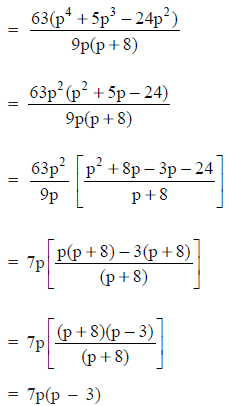Question 8: Simplify: –45p3 ÷ 9p2
Solution: We have –45p3 = (–1) * 3 * 3 * 5 * p * p * p
9p2 = 3 * 3 * p * p
∴ [–45p3] ÷ 9p2 = [(–1) * 3 * 3 * 5 * p * p * p] ÷ (3 * 3 * p * p)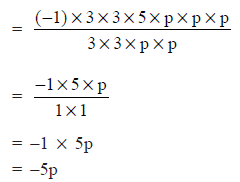Question 9: Simplify: 4x2y2(3z – 24) ÷ 36xy(z – 8)
Solution:
We have 3z – 24 = 3(z – 8)
∴ 4x2y2(3z –24) ÷ 36xy(z – 8)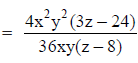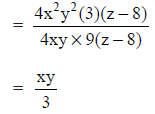Question 10: Divide: 81x3(50x2 – 98) by 27x2(5x + 7)
Solution:
We have 50x2 – 98 = 2(25x2 – 49)
= 2[(5x)2 – (7)2]
= 2[(5x + 7)(5x – 7)]            [Using a2 – b= (a + b)(a – b)]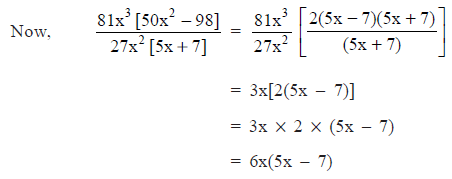The document Important Questions: Factorisation Notes | Study Mathematics (Maths) Class 8 - Class 8 is a part of the Class 8 Course Mathematics (Maths) Class 8.
All you need of Class 8 at this link: Class 8

## Mathematics (Maths) Class 8

187 videos|321 docs|48 tests
 Use Code STAYHOME200 and get INR 200 additional OFF

## Mathematics (Maths) Class 8

187 videos|321 docs|48 tests

Track your progress, build streaks, highlight & save important lessons and more!

,

,

,

,

,

,

,

,

,

,

,

,

,

,

,

,

,

,

,

,

,

;